# charge

Charge distribution on antenna or array surface

## Syntax

``charge(object,frequency)``
``c = charge(object,frequency)``
``[c,p] = charge(object,frequency)``
``charge(object,frequency,"dielectric")``
``c = charge(object,frequency,"dielectric")``
``c = charge(___,Name=Value)``

## Description

example

````charge(object,frequency)` calculates and plots the absolute value of the charge on the surface of an antenna or array at a specified frequency.```

example

````c = charge(object,frequency)` returns a vector of charges in C/m on the surface of an antenna or array at a specified frequency.```
````[c,p] = charge(object,frequency)` returns a vector of charges in C/m on the surface of an antenna or array at a specified frequency and the point at which the charge calculation was performed.```

example

````charge(object,frequency,"dielectric")` calculates and plots the absolute value of charge on the dielectric face of the antenna or array at a specified frequency.```
````c = charge(object,frequency,"dielectric")` returns the x, y, z components of the charge on the dielectric surface of an antenna or array at a specified frequency.```
````c = charge(___,Name=Value)` calculates the charge on the surface of an antenna or array using one or more name-value arguments. Specify name-value arguments after the input arguments.```

## Examples

collapse all

Calculate and plot the charge distribution on a bowtieTriangular antenna at 70 MHz frequency.

```h = bowtieTriangular; charge (h,70e6);```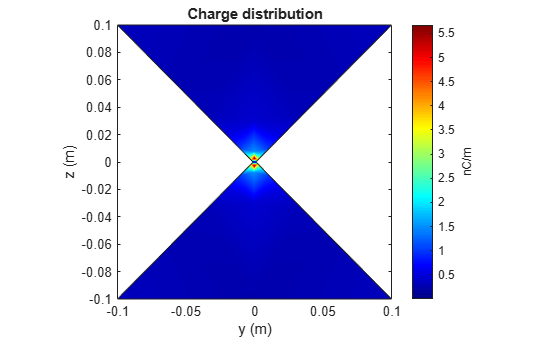Calculate charge distribution of linear array at 70 MHz frequency.

```h = linearArray; h.NumElements = 4; C = charge(h,70e6);```

Create a microstrip patch antenna using 'FR4' as the dielectric substrate.

```d = dielectric("FR4"); pm = patchMicrostrip(Length=75e-3, Width=37e-3, ... GroundPlaneLength=120e-3, GroundPlaneWidth=120e-3, ... Substrate=d)```
```pm = patchMicrostrip with properties: Length: 0.0750 Width: 0.0370 Height: 0.0060 Substrate: [1x1 dielectric] GroundPlaneLength: 0.1200 GroundPlaneWidth: 0.1200 PatchCenterOffset: [0 0] FeedOffset: [-0.0187 0] Conductor: [1x1 metal] Tilt: 0 TiltAxis: [1 0 0] Load: [1x1 lumpedElement] ```
`show(pm)`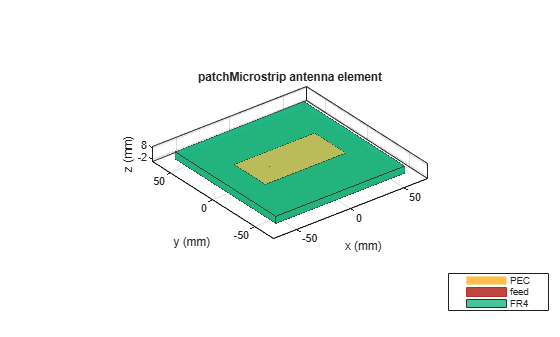Plot the charge distribution on the antenna at a frequency of 1.67 GHz.

```figure charge(pm,1.67e9,"dielectric")```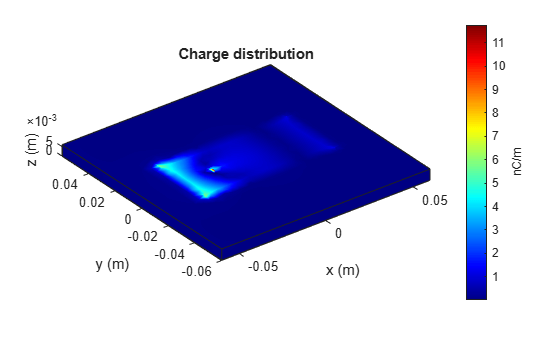Create a default pifa (planar inverted F antenna).

`ant = pifa;`

Visualize the charge distribution on the pifa antenna in log10 scale.

`charge(ant,1.75e9,Scale="log10")`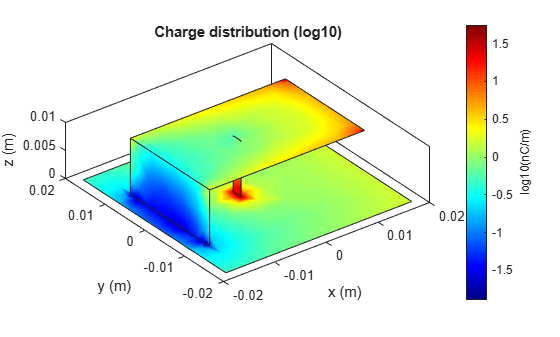Create a default cylindrical dielectric resonator antenna. Plot the charge distribution of the antenna at 1GHz with the `Slicer` argument set to `"on"`.

```ant = draCylindrical(ResonatorRadius=0.01); charge(ant,1e9,Slicer="on")```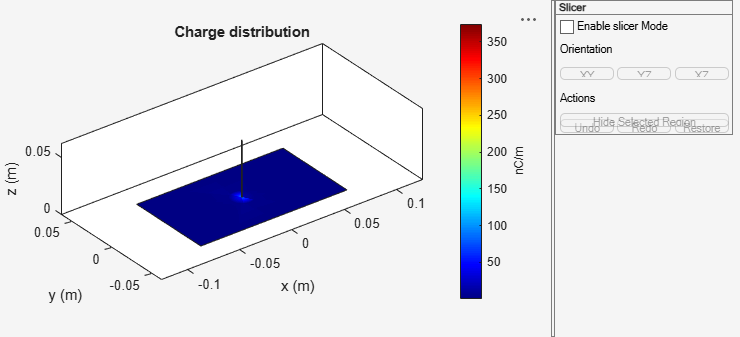Select Enable slicer Mode. Then select a plane for the slice under Orientation.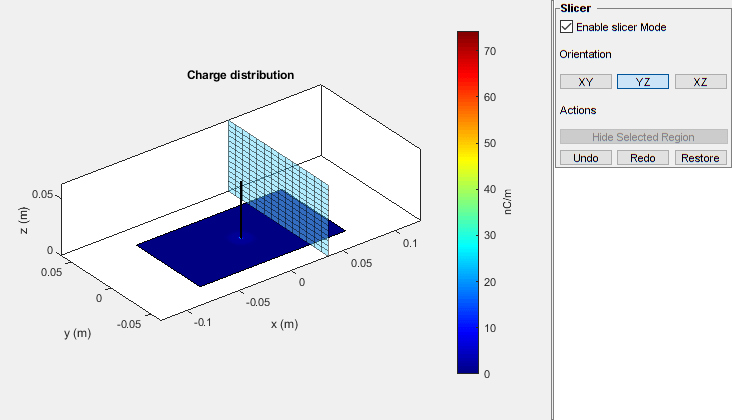Click on the plot and select a region to hide.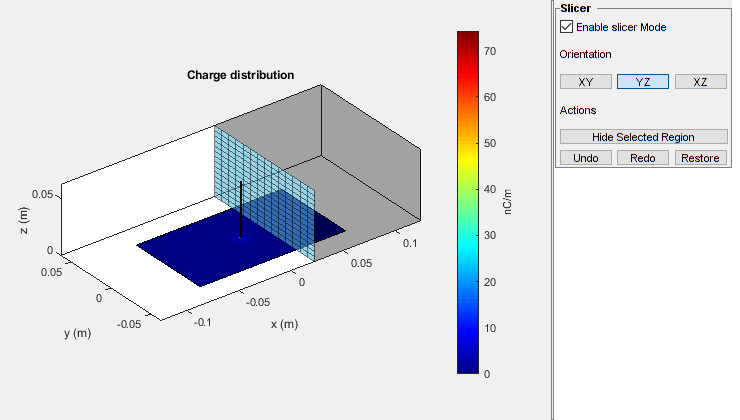Click Hide Selected Region to view the desired slice.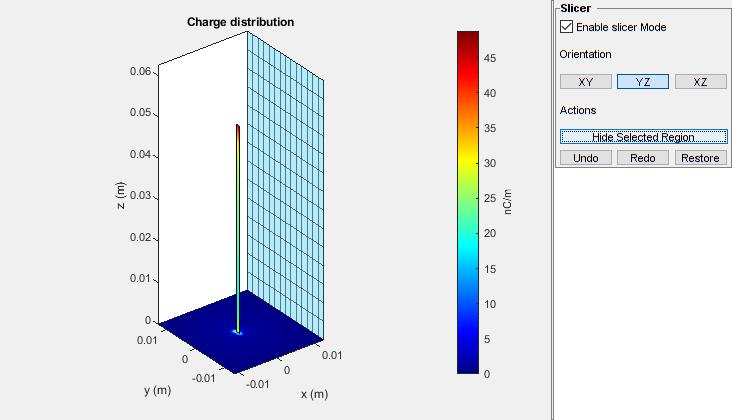## Input Arguments

collapse all

Antenna or array to calculate the charge distribution, specified as either of these:

Example: `dipole`

Example: `linearArray(Element=dipole)`

Frequency used to calculate charge distribution, specified as a scalar in Hz.

Example: 70e6

Data Types: `double`

### Name-Value Arguments

Specify optional pairs of arguments as `Name1=Value1,...,NameN=ValueN`, where `Name` is the argument name and `Value` is the corresponding value. Name-value arguments must appear after other arguments, but the order of the pairs does not matter.

Example: `Scale="log10"`

Before R2021a, use commas to separate each name and value, and enclose `Name` in quotes.

Example: `'Scale','log10'`

Layer to plot the charge distribution, specified as either `"metal"` or `"dielectric"`. Use this argument to choose between metal and dielectric layers and plot the charge distribution at the chosen layer.

Example: `"dielectric"`

Data Types: `string`

Scale of the charge distribution plot to visualize the charge distribution on the surface of the antenna or array, specified as a string or a logarithmic function. The default scale is `"linear"`.

Example: `"log10"`

Data Types: `string` | `function`

Option to enable or disable plot interactivity, specified as `"on"` or `"off"`, or as numeric or logical `1(true)` or `0(false)`. Set this argument to `1` or `"on"` to open the plot with the slicer panel, and to slice and view the desired cross section of the plot along the xy-, yz-, and xz- planes. Set this argument to `0` or `"off"` to open the plot without the slicer panel.

Example: `"on"`

Data Types: `string` | `logical`

## Output Arguments

collapse all

Complex charges, returned as a 1-by-n vector in C/m. This value is calculated for every triangle in the mesh or every dielectric tetrahedron face on the surface of an antenna or array.

Cartesian coordinates representing the center of each triangle in the mesh, returned as a 3-by-n real matrix.

## Version History

Introduced in R2015a